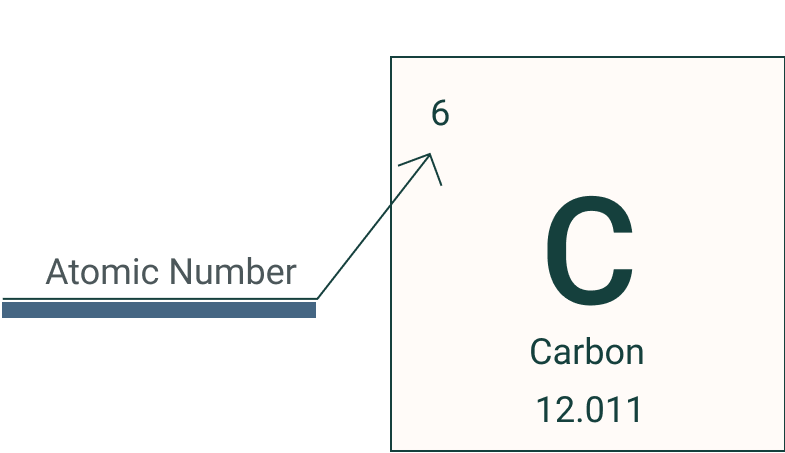Learn# Atomic Mass of the Elements

Beginner
1
m### Atomic mass refers to the mass of both the protons and neutrons and is always the larger of the two represented in the periodic table.

Atomic mass refers to the mass of both the protons and neutrons. This number is always the larger of the two represented in the periodic table. We can use this number to understand how many protons and neutrons there are in the nucleus. The example of Carbon and chlorine illustrates this well. Carbon has a mass listed as 12 and an atomic number of 6. Using this it can be seen that Carbon has 6 protons in its nucleus and subtracting the atomic number from the atomic mass (12-6) it can be seen that there are 6 neutrons in the nucleus.

For example, Oxygen has an atomic number of 8 indicating it has 8 protons in its nucleus. By looking at the mass number of 16 we can also deduce that there are 8 other particles in the nucleus, those 8 other particles are neutrons as neutrons and protons both have the same mass of 1. The mass of 16 is made up of 8 protons and 8 neutrons. This however does not consider the mass of the 8 electrons. An oxygen has 8 protons with 8 positive charges and 8 negatively charged electrons orbiting around it in shells.  The electron has such a small mass that we do not consider the mass of electrons in the atomic mass, the electron has a mass that is 0.00054 compared to the mass of protons and neutrons of 1.

###### Terms in section
Proton

A proton is a positive particle that makes up the atom in the nucleus with a positive charge

Neutron

A neutron is a neutral sub atomic particle that makes up the nucleus with the proton

Atomic mass

Atomic mass is the sum of the number of protons and neutrons in the nucleus

Atomic number

Atomic number is the number of protons in the nucleus

Electron

The electron is the smallest sub atomic particle that make up the atom. Has a negative charge and is located in shells that orbit the nucleus